## Recursion-

Recursion can be classified into following three types-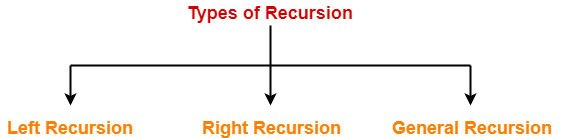1. Left Recursion
2. Right Recursion
3. General Recursion

## 1. Left Recursion-

• A production of grammar is said to have left recursion if the leftmost variable of its RHS is same as variable of its LHS.
• A grammar containing a production having left recursion is called as Left Recursive Grammar.

### Example-

S → Sa /

(Left Recursive Grammar)

• Left recursion is considered to be a problematic situation for Top down parsers.
• Therefore, left recursion has to be eliminated from the grammar.

## Elimination of Left Recursion

Left recursion is eliminated by converting the grammar into a right recursive grammar.

If we have the left-recursive pair of productions-

Aα / β

(Left Recursive Grammar)

where β does not begin with an A.

Then, we can eliminate left recursion by replacing the pair of productions with-

→ βA’

A’ → αA’ / ∈

(Right Recursive Grammar)

This right recursive grammar functions same as left recursive grammar.

## 2. Right Recursion-

• A production of grammar is said to have right recursion if the rightmost variable of its RHS is same as variable of its LHS.
• A grammar containing a production having right recursion is called as Right Recursive Grammar.

### Example-

S → aS /

(Right Recursive Grammar)

• Right recursion does not create any problem for the Top down parsers.
• Therefore, there is no need of eliminating right recursion from the grammar.

Also Read- Types of Recursive Grammar

## 3. General Recursion-

• The recursion which is neither left recursion nor right recursion is called as general recursion.

S → aSb /

## Problem-01:

Consider the following grammar and eliminate left recursion-

A → ABd / Aa / a

B → Be / b

## Solution-

The grammar after eliminating left recursion is-

A → aA’

A’ → BdA’ / aA’ /

B → bB’

B’ → eB’ /

## Problem-02:

Consider the following grammar and eliminate left recursion-

E → E + E / E x E / a

## Solution-

The grammar after eliminating left recursion is-

E → aA

A → +EA / xEA /

## Problem-03:

Consider the following grammar and eliminate left recursion-

E → E + T / T

T → T x F / F

F → id

## Solution-

The grammar after eliminating left recursion is-

E → TE’

E’ → +TE’ /

T → FT’

T’ → xFT’ /

F → id

## Problem-04:

Consider the following grammar and eliminate left recursion-

S → (L) / a

L → L , S / S

## Solution-

The grammar after eliminating left recursion is-

S → (L) / a

L → SL’

L’ → ,SL’ /

## Problem-05:

Consider the following grammar and eliminate left recursion-

S → S0S1S / 01

## Solution-

The grammar after eliminating left recursion is-

S → 01A

A → 0S1SA /

## Problem-06:

Consider the following grammar and eliminate left recursion-

S → A

A → Ad / Ae / aB / ac

B → bBc / f

## Solution-

The grammar after eliminating left recursion is-

S → A

A → aBA’ / acA’

A’ → dA’ / eA’ /

B → bBc / f

## Problem-07:

Consider the following grammar and eliminate left recursion-

A → AAα / β

## Solution-

The grammar after eliminating left recursion is-

A → βA’

A’ → AαA’ /

## Problem-08:

Consider the following grammar and eliminate left recursion-

A → Ba / Aa / c

B → Bb / Ab / d

## Solution-

This is a case of indirect left recursion.

### Step-01:

First let us eliminate left recursion from A → Ba / Aa / c

Eliminating left recursion from here, we get-

A → BaA’ / cA’

A’ → aA’ /

Now, given grammar becomes-

A → BaA’ / cA’

A’ → aA’ /

B → Bb / Ab / d

### Step-02:

Substituting the productions of A in B → Ab, we get the following grammar-

A → BaA’ / cA’

A’ → aA’ /

B → Bb / BaA’b / cA’b / d

### Step-03:

Now, eliminating left recursion from the productions of B, we get the following grammar-

A → BaA’ / cA’

A’ → aA’ /

B → cA’bB’ / dB’

B’ → bB’ / aA’bB’ /

This is the final grammar after eliminating left recursion.

## Problem-09:

Consider the following grammar and eliminate left recursion-

X → XSb / Sa / b

S → Sb / Xa / a

## Solution-

This is a case of indirect left recursion.

### Step-01:

First let us eliminate left recursion from X → XSb / Sa / b

Eliminating left recursion from here, we get-

X → SaX’ / bX’

X’ → SbX’ /

Now, given grammar becomes-

X → SaX’ / bX’

X’ → SbX’ /

S → Sb / Xa / a

### Step-02:

Substituting the productions of X in S → Xa, we get the following grammar-

X → SaX’ / bX’

X’ → SbX’ /

S → Sb / SaX’a / bX’a / a

### Step-03:

Now, eliminating left recursion from the productions of S, we get the following grammar-

X → SaX’ / bX’

X’ → SbX’ /

S → bX’aS’ / aS’

S’ → bS’ / aX’aS’ /

This is the final grammar after eliminating left recursion.

## Problem-10:

Consider the following grammar and eliminate left recursion-

S → Aa / b

A → Ac / Sd /

## Solution-

This is a case of indirect left recursion.

### Step-01:

First let us eliminate left recursion from S → Aa / b

This is already free from left recursion.

### Step-02:

Substituting the productions of S in A → Sd, we get the following grammar-

S → Aa / b

A → Ac / Aad / bd /

### Step-03:

Now, eliminating left recursion from the productions of A, we get the following grammar-

S → Aa / b

A → bdA’ / A’

A’ → cA’ / adA’ /

This is the final grammar after eliminating left recursion.

To gain better understanding about Left Recursion Elimination,

Watch this Video Lecture

Next Article- Types of Grammars

Get more notes and other study material of Theory of Automata and Computation.

Watch video lectures by visiting our YouTube channel LearnVidFun.

## Relationship Between Left Recursion, Left Factoring & Ambiguity-

 There is no relationship between Left Recursion, Left Factoring and Ambiguity of Grammar.

• All the three concepts are independent and has nothing to do with each other.
• The presence or absence of left recursion does not impact left factoring and ambiguity anyhow.
• The presence or absence of left factoring does not impact left recursion and ambiguity anyhow.
• The presence or absence of ambiguity does not impact left recursion and left factoring anyhow.

The following examples support this fact-

### Example-01: Ambiguous Grammar With Left Factoring-

Consider the following grammar-

S → aS / a / ∈

Clearly, this grammar has left factoring.

Now, let us draw parse trees for the string w = a-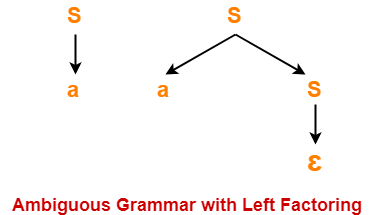Clearly,

• Two different parse trees exist for the string w = a.
• Therefore, the grammar is ambiguous.

### Example-02: Unambiguous Grammar With Left Factoring-

Consider the following grammar-

S → aA / aB

A → a

B → b

Clearly, this grammar has left factoring.

The language generated by this grammar consists of only two strings L(G) = { aa , ab}.

Now, let us draw parse trees for these strings-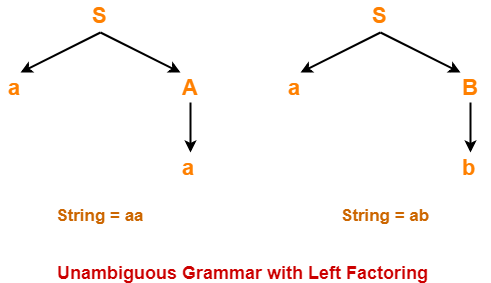Clearly,

• A unique parse tree exists for both the strings.
• Therefore, the grammar is unambiguous.

### Example-03: Ambiguous Grammar With Left Recursion-

Consider the following grammar-

S → SS / ∈

Clearly, this grammar has left recursion.

Now, let us draw parse trees for the string w = ∈-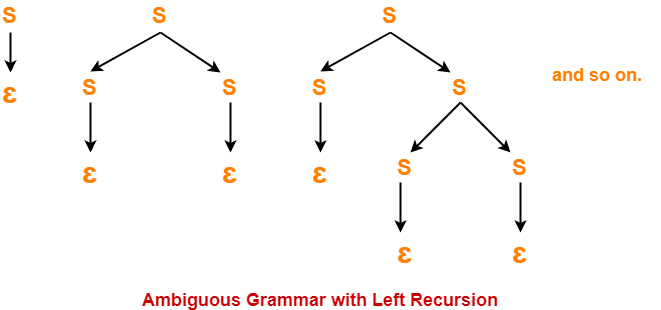Clearly,

• Infinite parse trees exist for the string w = ∈.
• Therefore, the grammar is ambiguous.

### Example-04: Unambiguous Grammar With Left Recursion-

Consider the following grammar-

S → Sa / ∈

Clearly, this grammar has left recursion.

A unique parse tree exists for all the strings that can be generated from the grammar.

Therefore, the grammar is unambiguous.

### Example-05: Ambiguous Grammar Without Left Recursion & Without Left Factoring-

Consider the following grammar-

S → aA / Ba

A → a

B → a

Clearly, this grammar has neither left recursion nor left factoring.

Now, let us draw parse trees for the string w = aa-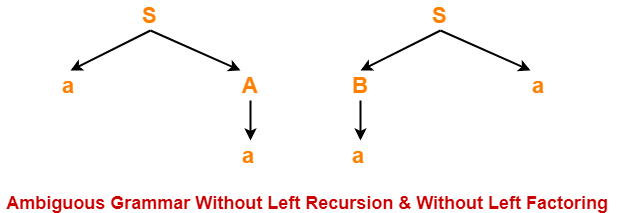Clearly,

• Two different parse trees exist for the string w = aa.
• Therefore, the grammar is ambiguous.

### Example-06: Unambiguous Grammar With Both Left Recursion & Left Factoring-

Consider the following grammar-

S → Sa / ɛ / bB / bD

B → b

D → d

Clearly, this grammar has both left recursion and left factoring.

A unique parse tree exists for all the strings that can be generated from the grammar.

Therefore, the grammar is unambiguous.

To gain better understanding about relationship between left recursion, left factoring and ambiguity-

Watch this Video LectureNext Article- Calculating First and Follow

Get more notes and other study material of Compiler Design.

Watch video lectures by visiting our YouTube channel LearnVidFun.